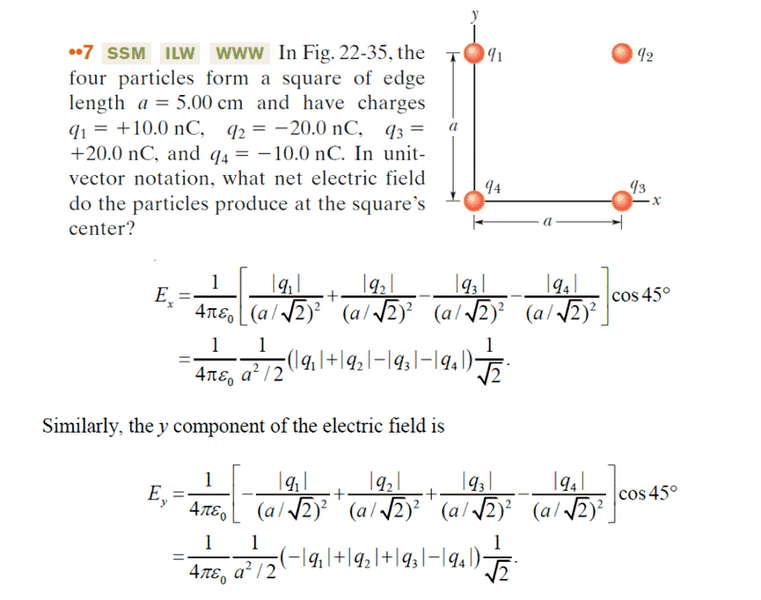# Another electric field question

• cros0
The x and y components of the electric field vectors are computed by finding the magnitude of each vector and multiplying by cos(45) or cos(-45). This will give you the x and y components in the same coordinate system as the charge.f

#### cros0The first part of the above image is the question from the text, and below that is information from the solutions manual. I understand why we broke the components up into the x and y, I understand how we triangulated the distance between the center of our coordinate system and the charge to get a/sqrt(2). What I don't understand why we are multiplying by the cos45 in both x and y. Anyone have any ideas?

Hi cros0. Please remember to use the formatting template provided in the edit window when you create a homework help request. This is in the PF rules.

Did you sketch in the electric field vectors that each charge produces at the center of the square? What directions do they lie along? How do those directions compare to the implied x and y axes directions?

Hi cros0. Please remember to use the formatting template provided in the edit window when you create a homework help request. This is in the PF rules.

Did you sketch in the electric field vectors that each charge produces at the center of the square? What directions do they lie along? How do those directions compare to the implied x and y axes directions?

Hello and thanks for the response. I did not understand that we had to deal with vectors in this problem. I have reviewed the text and I understand now. Finding the magnitude of the vectors of both x and y components is going to require multiplying by cos45 or cos(-45) which will give the same answer. I am going to have to go over the previous chapter again because I think I missed this point.

Hello and thanks for the response. I did not understand that we had to deal with vectors in this problem. I have reviewed the text and I understand now. Finding the magnitude of the vectors of both x and y components is going to require multiplying by cos45 or cos(-45) which will give the same answer. I am going to have to go over the previous chapter again because I think I missed this point.
Yup. You need to break the individual vectors into their components in some assumed coordinate system in order to add together their individual contributions. Essentially this is an exercise in vector addition.